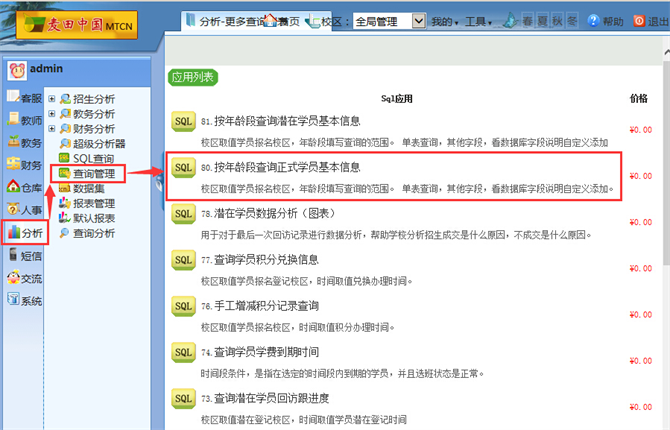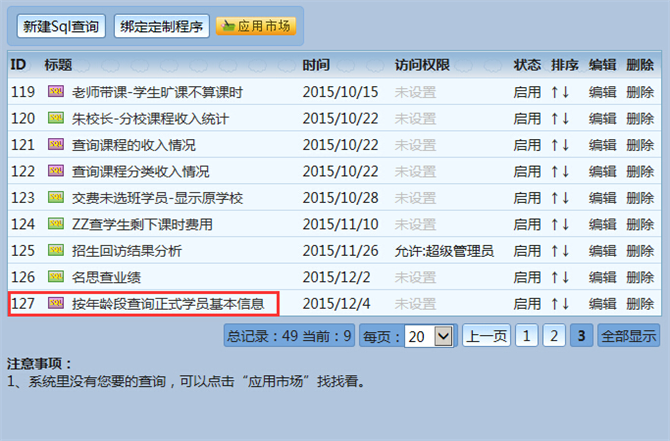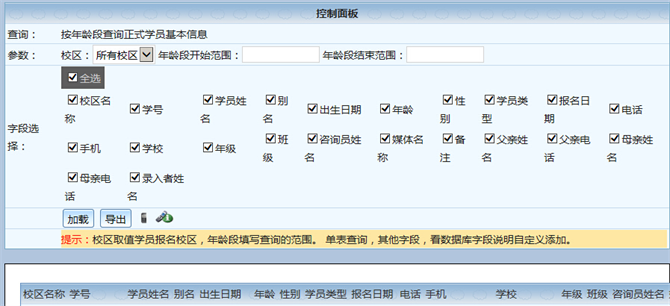查询篇-按年龄段查询正式学员基本信息-麦田培训学校管理系统

 2015年12月04日 05:18 点击率：4225

【关于麦田查询】

【相关代码-SQL版】

select
Student.SchoolName as 校区名称,
Student.StudentID as 学号,
Student.StudentName as 学员姓名,
Student.Byname as 别名,
Student.Birthday as 出生日期,
DateDiff(year,Student.Birthday,getdate()) as 年龄,
GetSex(Student.Sex) as 性别,
GetStudentType(Student.StudentType) as 学员类型,
Student.DateAndTime as 报名日期,
Student.Tel as 电话,
Student.MobileTel as 手机,
Student.School as 学校,
Student.Class as 班级,
Student.ConsultantName as 咨询员姓名,
Student.MediaName as 媒体名称,
Student.Remark as 备注,
Student.FatherName as 父亲姓名,
Student.FatherMobileTel as 父亲电话,
Student.MotherName as 母亲姓名,
Student.MotherMobileTel as 母亲电话,
Student.TrueName as 录入者姓名
from Student
where
Student.SchoolID in ( {@SchoolID:校区} )
and DateDiff(year,Student.Birthday,getdate()) >= {@Key:年龄段开始范围}
and DateDiff(year,Student.Birthday,getdate()) <= {@Key1:年龄段结束范围}

【相关代码-AC版】

select
Student.SchoolName as 校区名称,
Student.StudentID as 学号,
Student.StudentName as 学员姓名,
Student.Byname as 别名,
Student.Birthday as 出生日期,
year(now())-year(Student.Birthday) as 年龄,
GetSex(Student.Sex) as 性别,
GetStudentType(Student.StudentType) as 学员类型,
Student.DateAndTime as 报名日期,
Student.Tel as 电话,
Student.MobileTel as 手机,
Student.School as 学校,
Student.Class as 班级,
Student.ConsultantName as 咨询员姓名,
Student.MediaName as 媒体名称,
Student.Remark as 备注,
Student.FatherName as 父亲姓名,
Student.FatherMobileTel as 父亲电话,
Student.MotherName as 母亲姓名,
Student.MotherMobileTel as 母亲电话,
Student.TrueName as 录入者姓名
from Student
where
Student.SchoolID in ( {@SchoolID:校区} )
and year(now())-year(Student.Birthday) >= CInt( {@Key1:年龄段开始范围} )
and year(now())-year(Student.Birthday) <= CInt( {@Key2:年龄段结束范围} )

【相关截图】【关于麦田】